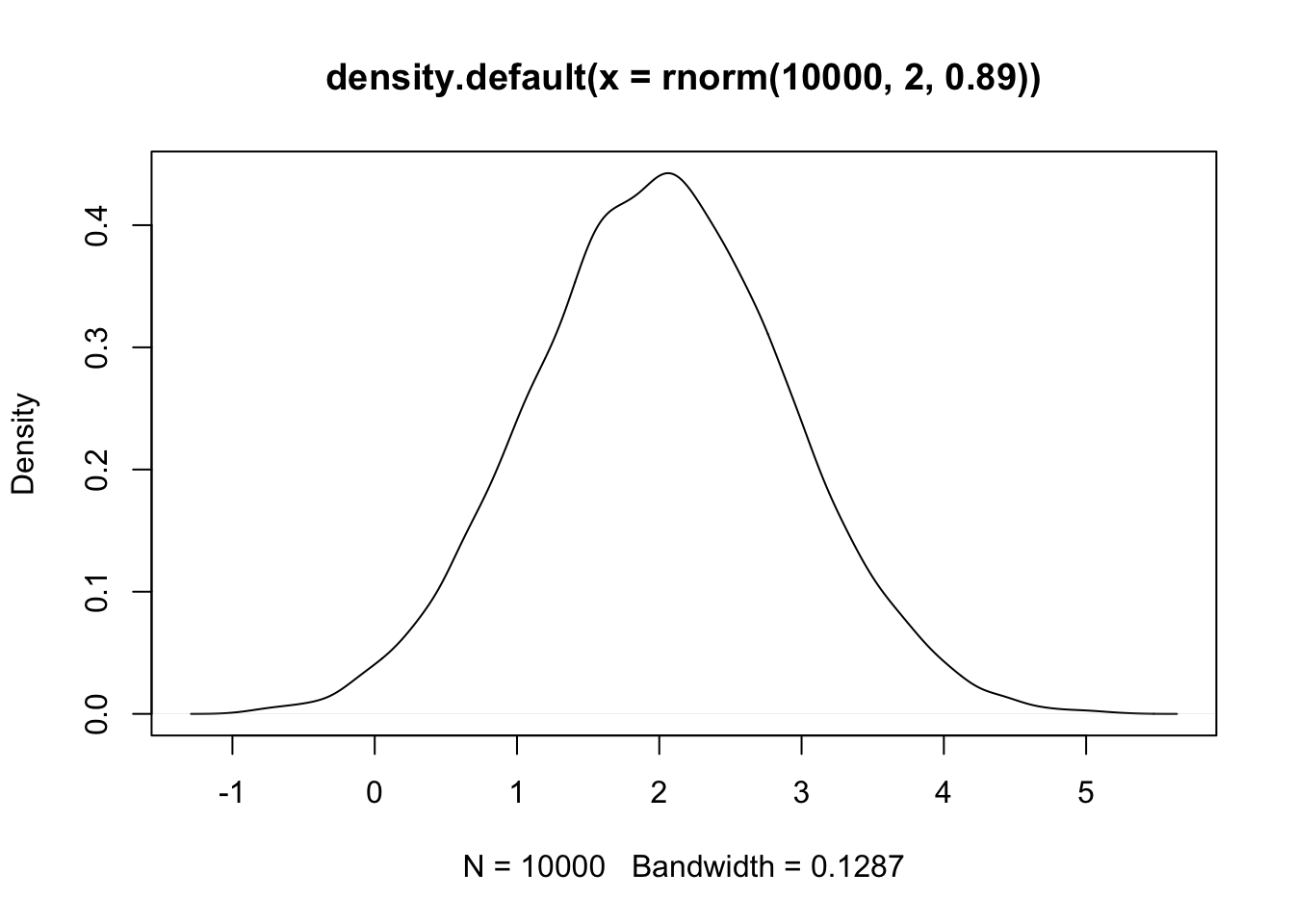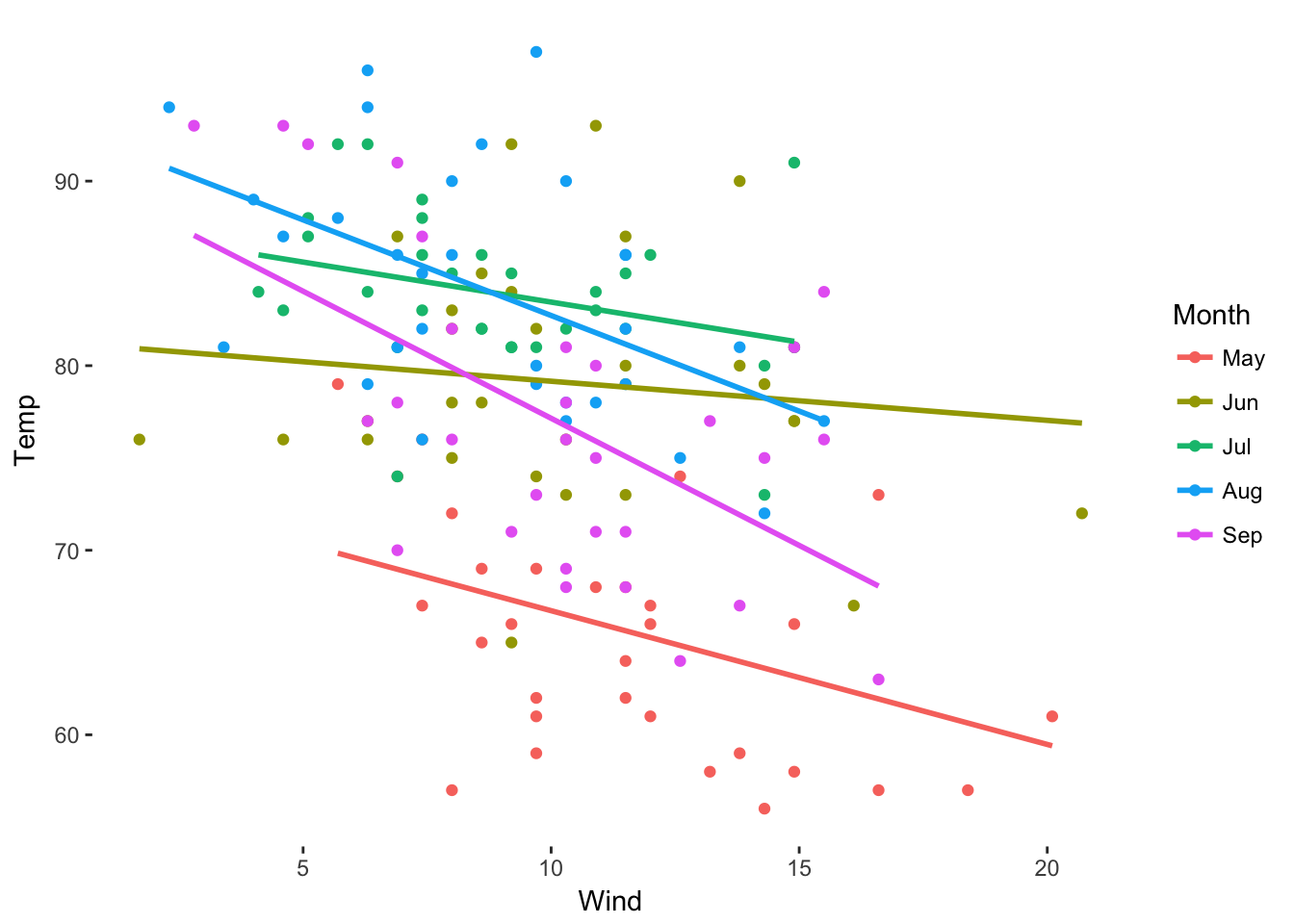All examples in this document use default R datasets.

• Create a 10 length vector that goes from 0 to 9, but replace the 5 with 15
x <- 0:9
x <- 15
x <- c(0:4, 15, 6:9) # do it in one line
x
##    0  1  2  3  4 15  6  7  8  9
• Multiply a vector by a scalar
45:57 * .42
##   18.90 19.32 19.74 20.16 20.58 21.00 21.42 21.84 22.26 22.68 23.10
##  23.52 23.94
• Matrix multiply a vector by a $$10 \times 4$$ matrix of draws from a Beta(2,1) distribution
1:10 %*% matrix(rbeta(400, 2, 1), nrow = 10, ncol = 4)
##       [,1]  [,2]  [,3] [,4]
## [1,] 33.02 34.13 39.78 34.4
• Subset the Seatbelts data to include only the drivers, rear, PetrolPrice, and law columns
data.frame(Seatbelts[, c('drivers', 'rear', 'PetrolPrice', 'law')])
• Subset the CO2 data to include only observations where the plant’s CO$$_2$$ uptake rate is less than or equal to 15
CO2[which(CO2$uptake <= 15), ] • Sort the mtcars data in ascending order by cylinders and miles per gallon mtcars[order(mtcars$cyl, mtcars$mpg), ] • Generate 10000 draws from a $$\mathcal{N}(2, .89)$$ distribution, and plot their density plot(density(rnorm(1e4, 2, .89)))• Call the invlogit() function from arm without loading the package arm::invlogit(.034) ##  0.5085 • Using the mtcars data, fit a linear model that explains variation in miles per gallon as a function of number of cylinders, displacement, and horsepower. Extract the coefficients, standard error, and R$$^2$$ from the model. m1 <- lm(mpg ~ cyl + disp + hp, data = mtcars) coef(m1) ## (Intercept) cyl disp hp ## 34.18492 -1.22742 -0.01884 -0.01468 sqrt(diag(vcov(m1))) ## (Intercept) cyl disp hp ## 2.59078 0.79728 0.01040 0.01465 summary(m1)$r.squared
##  0.7679
• Use the Titanic data to fit a model that explains whether a passenger survived the ship’s sinking as a function of their sex, age, and passenger class, but use a probit link function. What is the difference in coefficient estimates between this model and one using the canonical logit link function?
coef(glm(Survived ~ Class + Sex + Age, data = Titanic, family = binomial(link = 'probit'))) -
coef(glm(Survived ~ Class + Sex + Age, data = Titanic, family = binomial(link = 'logit')))
## (Intercept)    Class2nd    Class3rd   ClassCrew   SexFemale    AgeAdult
##  -2.902e-16   3.942e-16   4.920e-16   6.943e-16  -2.156e-16   2.214e-16
• Write a loop that generates 1000 draws from a $$\mathcal{N}(-2.5, 4)$$ distribution, and then records their mean. Run the loop for 10000 iterations and report the mean of the means.
x <- numeric()
for (i in 1:1e4) {

x[i] <- mean(rnorm(1e3, -2.5, 4))

}
mean(x)
##  -2.501
• Write a mean function
my.mean <- function(x) {

sum(x) / length(x)

}

my.mean(1:7)
##  4
• Write a mean function that can handle NA values
my.mean.NA <- function(x) {

x <- na.omit(x)
sum(x) / length(x)

}

my.mean.NA(c(NA, 1:7, NA))
##  4
• Write a function that accepts a vector, squares even integers, and square roots all other numbers
myfunc <- function(x) {

for (i in 1:length(x)) {

if (x[i] %% 2 == 0) {

x[i] <- x[i]^2

} else {

x[i] <- sqrt(x[i])

}

}

x

}

myfunc(seq(1, 6, by = .5))
##    1.000  1.225  4.000  1.581  1.732  1.871 16.000  2.121  2.236  2.345
##  36.000
• Use the airquality data to plot wind speed against temperature. Use separate colors for observations in each month, and include a linear fit line for each month.
library(ggplot2)
ggplot(data = airquality, aes(x = Wind, y = Temp, color = as.factor(Month))) +
geom_point() +
geom_smooth(method = 'lm', se = F) +
labs(color = 'Month') +
scale_color_discrete(labels = c('May', 'Jun', 'Jul', 'Aug', 'Sep')) +
theme_bw() +
theme(legend.position = 'right',
plot.background = element_blank(),
panel.grid.minor = element_blank(),
panel.grid.major = element_blank(),
panel.border = element_blank())